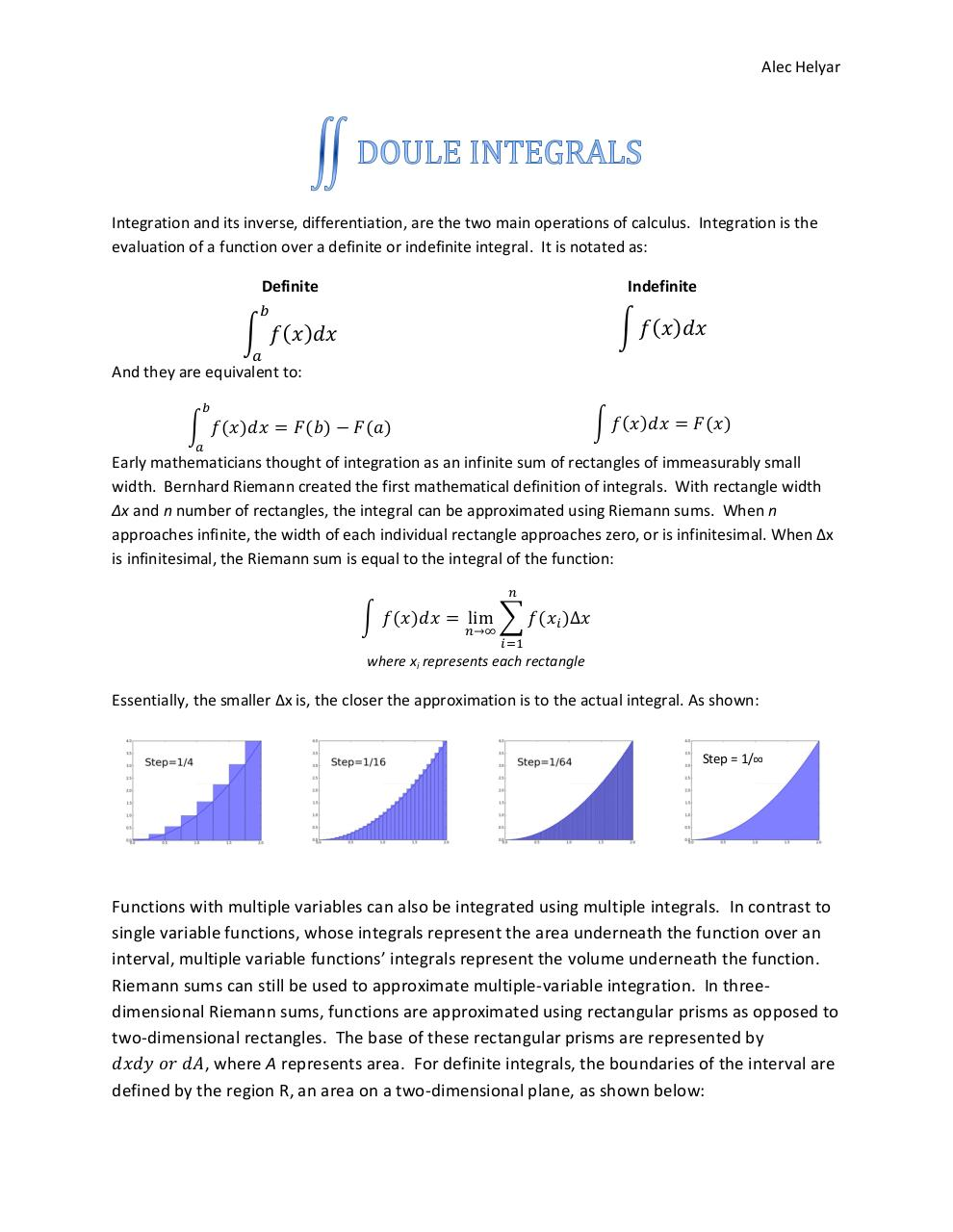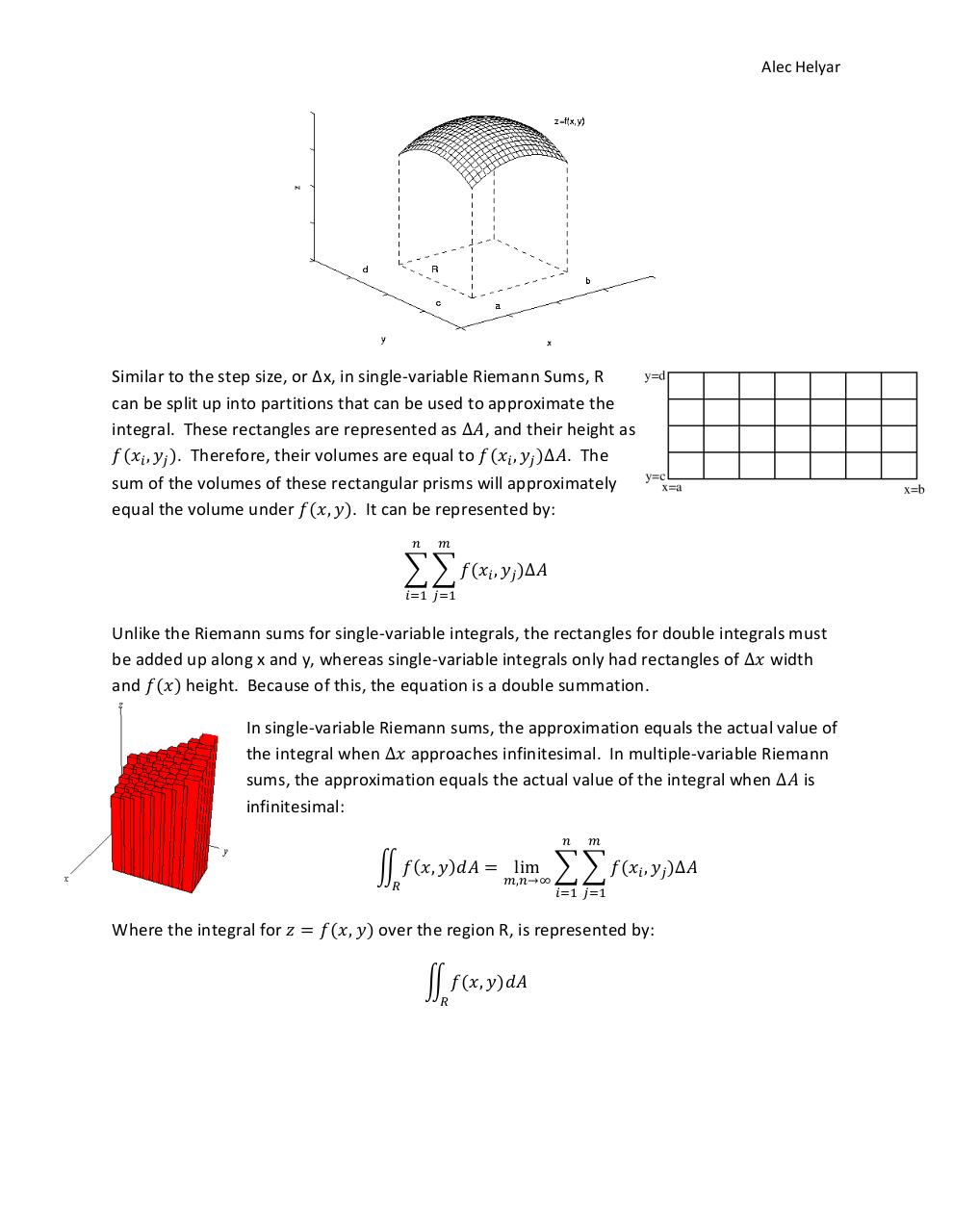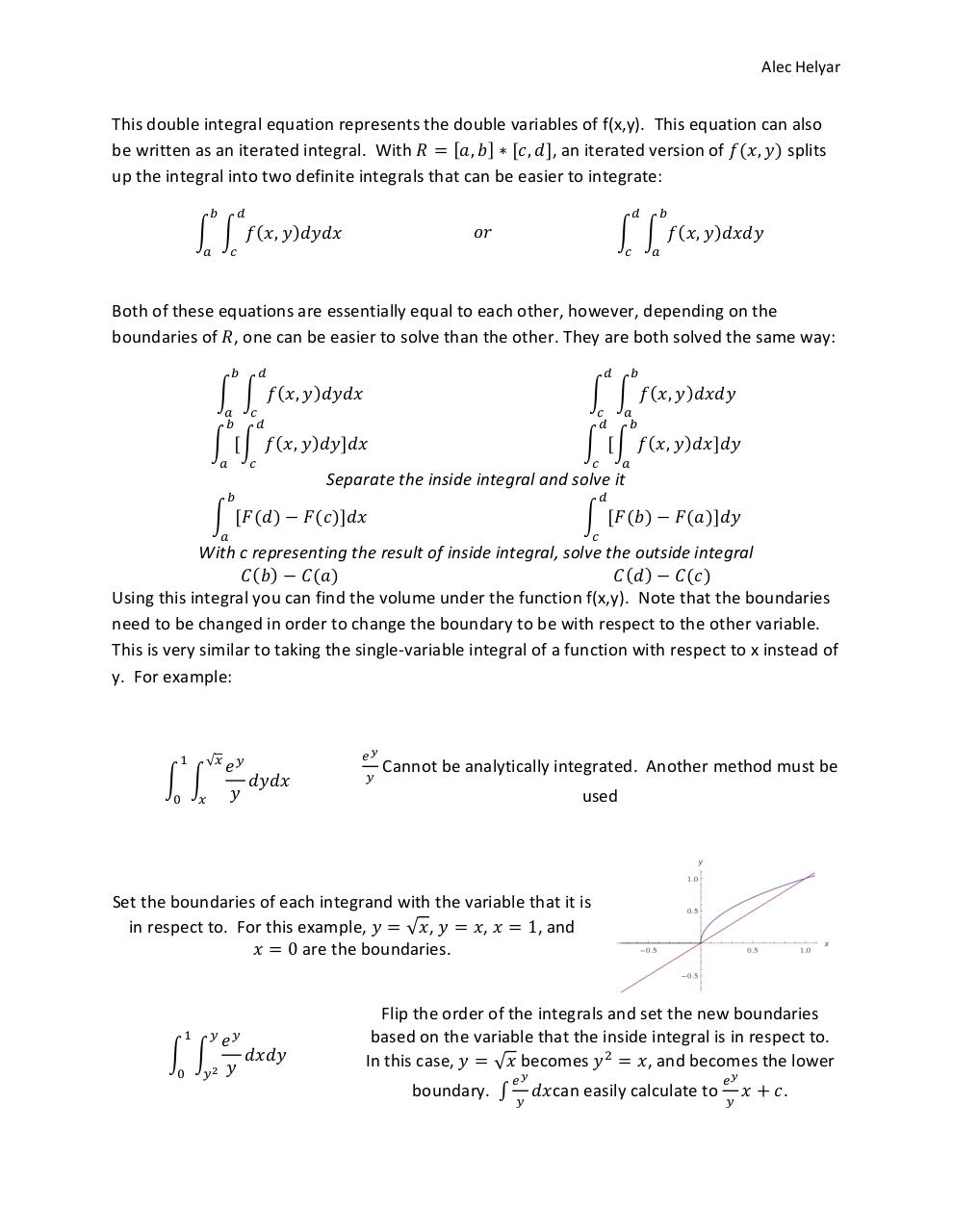# Calculus at it's finest .pdf

### File information

Original filename: Calculus at it's finest.pdf
Author: Alec

This PDF 1.5 document has been generated by MicrosoftÂ® Word 2010, and has been sent on pdf-archive.com on 02/06/2014 at 05:06, from IP address 174.97.x.x. The current document download page has been viewed 805 times.
File size: 496 KB (4 pages).
Privacy: public file

Calculus at it's finest.pdf (PDF, 496 KB)

### Document preview

Alec Helyar

Integration and its inverse, differentiation, are the two main operations of calculus. Integration is the
evaluation of a function over a definite or indefinite integral. It is notated as:
Definite

Indefinite

∫ ( )

( )

And they are equivalent to:

( )

( )

∫ ( )

( )

( )

Early mathematicians thought of integration as an infinite sum of rectangles of immeasurably small
width. Bernhard Riemann created the first mathematical definition of integrals. With rectangle width
∆x and n number of rectangles, the integral can be approximated using Riemann sums. When n
approaches infinite, the width of each individual rectangle approaches zero, or is infinitesimal. When ∆x
is infinitesimal, the Riemann sum is equal to the integral of the function:

∫ ( )

∑ ( )

where xi represents each rectangle

Essentially, the smaller ∆x is, the closer the approximation is to the actual integral. As shown:

Step = 1/∞

Functions with multiple variables can also be integrated using multiple integrals. In contrast to
single variable functions, whose integrals represent the area underneath the function over an
interval, multiple variable functions’ integrals represent the volume underneath the function.
Riemann sums can still be used to approximate multiple-variable integration. In threedimensional Riemann sums, functions are approximated using rectangular prisms as opposed to
two-dimensional rectangles. The base of these rectangular prisms are represented by
, where A represents area. For definite integrals, the boundaries of the interval are
defined by the region R, an area on a two-dimensional plane, as shown below:

Alec Helyar

Similar to the step size, or ∆x, in single-variable Riemann Sums, R
can be split up into partitions that can be used to approximate the
integral. These rectangles are represented as , and their height as
(
). Therefore, their volumes are equal to (
) . The
sum of the volumes of these rectangular prisms will approximately
equal the volume under (
). It can be represented by:
∑∑ (

)

Unlike the Riemann sums for single-variable integrals, the rectangles for double integrals must
be added up along x and y, whereas single-variable integrals only had rectangles of
width
and ( ) height. Because of this, the equation is a double summation.
In single-variable Riemann sums, the approximation equals the actual value of
the integral when
approaches infinitesimal. In multiple-variable Riemann
sums, the approximation equals the actual value of the integral when
is
infinitesimal:
∬ (
Where the integral for

(

)

∑∑ (

) over the region R, is represented by:
∬ (

)

)

Alec Helyar

This double integral equation represents the double variables of f(x,y). This equation can also
[
] [ ], an iterated version of (
be written as an iterated integral. With
) splits
up the integral into two definite integrals that can be easier to integrate:
∫ ∫

(

)

∫ ∫

(

)

Both of these equations are essentially equal to each other, however, depending on the
boundaries of , one can be easier to solve than the other. They are both solved the same way:
∫ ∫

(

)

∫ [∫

(

)

]

∫ ∫

(

)

∫ [∫

(

)

]

Separate the inside integral and solve it
∫ [ ( )

( )]

∫ [ ( )

( )]

With c representing the result of inside integral, solve the outside integral
( )
( )
( )
( )
Using this integral you can find the volume under the function f(x,y). Note that the boundaries
need to be changed in order to change the boundary to be with respect to the other variable.
This is very similar to taking the single-variable integral of a function with respect to x instead of
y. For example:

∫ ∫

Cannot be analytically integrated. Another method must be
used

Set the boundaries of each integrand with the variable that it is
in respect to. For this example,
,
, and
√ ,
are the boundaries.

∫ ∫

Flip the order of the integrals and set the new boundaries
based on the variable that the inside integral is in respect to.
In this case,
, and becomes the lower
√ becomes
boundary. ∫

can easily calculate to

.

Alec Helyar#### HTML Code

Copy the following HTML code to share your document on a Website or Blog

#### QR Code### Related keywords